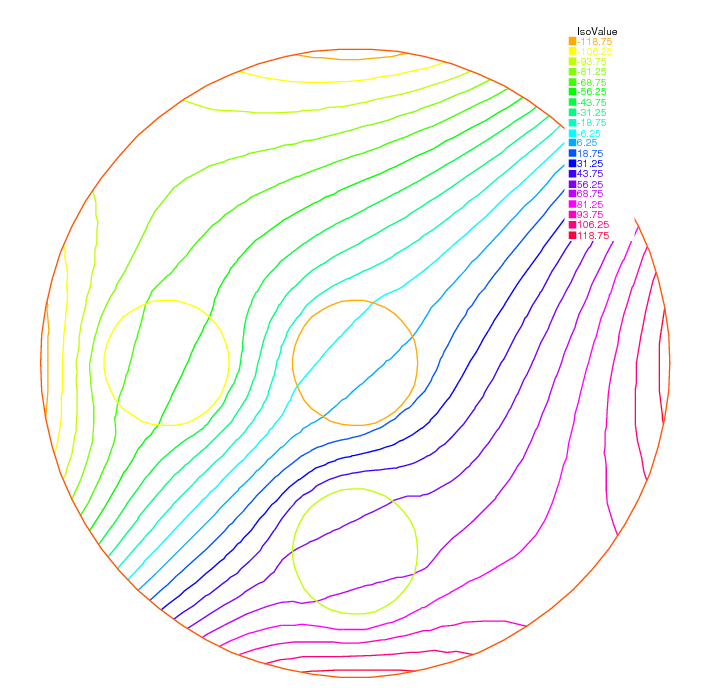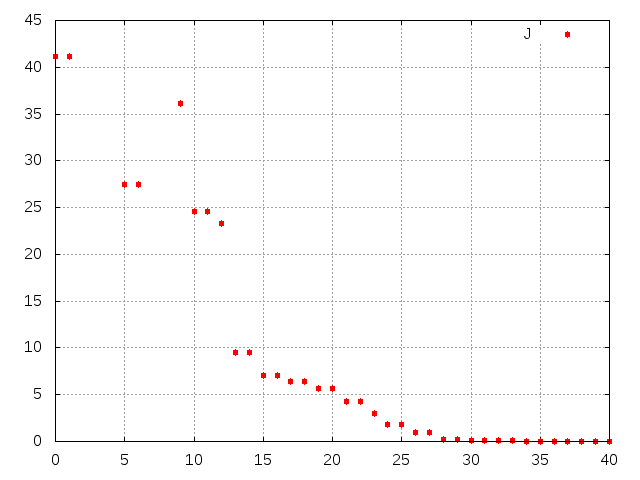FreeFEM Documentation on GitHub

stars - forks

# Optimal Control

Thanks to the function BFGS it is possible to solve complex nonlinear optimization problem within FreeFEM. For example consider the following inverse problem

$\begin{split}\begin{array}{rcl} \min_{b, c, d\in R}J &=& \int_E(u-u_d)^2\\ -\nabla(\kappa(b, c, d)\cdot\nabla u) &=& 0\\ u|_\Gamma &=& u_\Gamma \end{array}\end{split}$

where the desired state $$u_d$$, the boundary data $$u_\Gamma$$ and the observation set $$E\subset\Omega$$ are all given. Furthermore let us assume that:

$\kappa(x)=1+bI_B(x)+cI_C(x)+dI_D(x)\quad\forall x\in\Omega$

where $$B,C,D$$ are separated subsets of $$\Omega$$.

To solve this problem by the quasi-Newton BFGS method we need the derivatives of $$J$$ with respect to $$b,c,d$$. We self explanatory notations, if $$\delta b,\delta c,\delta d$$ are variations of $$b,c,d$$ we have:

$\begin{split}\begin{array}{rcl} \delta J &\approx& 2\int_E(u-u_d)\delta u\\ -\nabla(\kappa\cdot\nabla\delta u) &\approx& \nabla(\delta\kappa\cdot\nabla u)\\ \delta u|_\Gamma &=& 0 \end{array}\end{split}$

Obviously $$J'_b$$ is equal to $$\delta J$$ when $$\delta b=1,\delta c=0,\delta d=0$$, and so on for $$J'_c$$ and $$J'_d$$.

All this is implemented in the following program:

 1// Mesh
2border aa(t=0, 2*pi){x=5*cos(t); y=5*sin(t);};
3border bb(t=0, 2*pi){x=cos(t); y=sin(t);};
4border cc(t=0, 2*pi){x=-3+cos(t); y=sin(t);};
5border dd(t=0, 2*pi){x=cos(t); y =-3+sin(t);};
6
7mesh th = buildmesh(aa(70) + bb(35) + cc(35) + dd(35));
8
9// Fespace
10fespace Vh(th, P1);
11Vh Ib=((x^2+y^2)<1.0001),
12   Ic=(((x+3)^2+ y^2)<1.0001),
13   Id=((x^2+(y+3)^2)<1.0001),
14   Ie=(((x-1)^2+ y^2)<=4),
15   ud, u, uh, du;
16
17// Problem
18real[int] z(3);
19problem A(u, uh)
20   = int2d(th)(
21        (1+z*Ib+z*Ic+z*Id)*(dx(u)*dx(uh) + dy(u)*dy(uh))
22   )
23   + on(aa, u=x^3-y^3)
24   ;
25
26// Solve
27z=2; z=3; z=4;
28A;
29ud = u;
30
31ofstream f("J.txt");
32func real J(real[int] & Z){
33   for (int i = 0; i < z.n; i++)
34      z[i] =Z[i];
35   A;
36   real s = int2d(th)(Ie*(u-ud)^2);
37   f << s << " ";
38   return s;
39}
40
41// Problem BFGS
42real[int] dz(3), dJdz(3);
43problem B (du, uh)
44   = int2d(th)(
45        (1+z*Ib+z*Ic+z*Id)*(dx(du)*dx(uh) + dy(du)*dy(uh))
46   )
47   + int2d(th)(
48        (dz*Ib+dz*Ic+dz*Id)*(dx(u)*dx(uh) + dy(u)*dy(uh))
49   )
50   +on(aa, du=0)
51   ;
52
53func real[int] DJ(real[int] &Z){
54   for(int i = 0; i < z.n; i++){
55      for(int j = 0; j < dz.n; j++)
56         dz[j] = 0;
57      dz[i] = 1;
58      B;
59      dJdz[i] = 2*int2d(th)(Ie*(u-ud)*du);
60   }
61   return dJdz;
62}
63
64real[int] Z(3);
65for(int j = 0; j < z.n; j++)
66   Z[j]=1;
67
68BFGS(J, DJ, Z, eps=1.e-6, nbiter=15, nbiterline=20);
69cout << "BFGS: J(z) = " << J(Z) << endl;
70for(int j = 0; j < z.n; j++)
71   cout << z[j] << endl;
72
73// Plot
74plot(ud, value=1, ps="u.eps");


In this example the sets $$B,C,D,E$$ are circles of boundaries $$bb,cc,dd,ee$$ and the domain $$\Omega$$ is the circle of boundary $$aa$$.

The desired state $$u_d$$ is the solution of the PDE for $$b=2,c=3,d=4$$. The unknowns are packed into array $$z$$.

Note

It is necessary to recopy $$Z$$ into $$z$$ because one is a local variable while the other one is global.

The program found $$b=2.00125,c=3.00109,d=4.00551$$.

Fig. 40 and Fig. 41 show $$u$$ at convergence and the successive function evaluations of $$J$$.Fig. 40 Level line of $$u$$.Fig. 41 Successive evaluations of $$J$$ by BFGS (5 values above 500 have been removed for readability)

Optimal control

Note that an adjoint state could have been used. Define $$p$$ by:

$\begin{split}\begin{array}{rcl} -\nabla\cdot(\kappa\nabla p) &=& 2I_E(u-u_d)\\ p|_\Gamma &=& 0 \end{array}\end{split}$

Consequently:

$\begin{split}\begin{array}{rcl} \delta J &=& -\int_{\Omega}(\nabla\cdot(\kappa\nabla p))\delta u\nonumber\\ &=& \int_\Omega(\kappa\nabla p\cdot\nabla\delta u)\\ &=&-\int_\Omega(\delta\kappa\nabla p\cdot\nabla u) \end{array}\end{split}$

Then the derivatives are found by setting $$\delta b=1, \delta c=\delta d=0$$ and so on:

$\begin{split}\begin{array}{rcl} J'_b&=&-\int_B \nabla p\cdot\nabla u\\ J'_c&=&-\int_C \nabla p\cdot\nabla u\\ J'_d&=&-\int_D \nabla p\cdot\nabla u \end{array}\end{split}$

Note

As BFGS stores an $$M\times M$$ matrix where $$M$$ is the number of unknowns, it is dangerously expensive to use this method when the unknown $$x$$ is a Finite Element Function. One should use another optimizer such as the NonLinear Conjugate Gradient NLCG (also a key word of FreeFEM).

Table of content